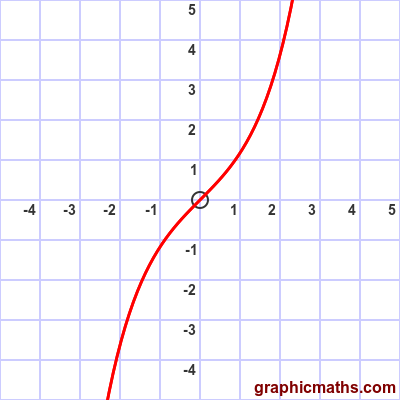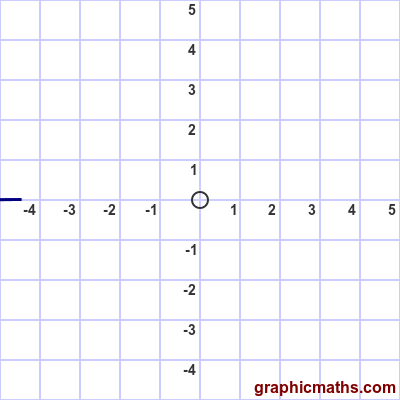# sinh function

By Martin McBride, 2020-09-10
Tags: sinh hyperbolic function
Categories: hyperbolic functions pure mathematics

The sinh function is a hyperbolic function. It is also known as the hyperbolic sine function.

Here is a video that explains sinh, cosh and tanh:

## Equation and graph

The sinh function is defined as:

$$\sinh{x} = \frac{e^{x}-e^{-x}}{2}$$

Here is a graph of the function:## sinh as average of two exponentials

The sinh function can be interpreted as the average of two functions, $e^{x}$ and $-e^{-x}$. This animation illustrates this:## Other forms of the equation

If we multiply the top and bottom of the original equation for the sinh function by $e^{x}$ (see below) we get:

$$\sinh{x} = \frac{e^{2x}-1}{2e^{x}}$$

Alternatively, if we multiply the top and bottom of the original equation for the sinh function by $e^{-x}$ we get:

$$\sinh{x} = \frac{1-e^{-2x}}{2e^{-x}}$$

## Derivation of other forms

To see how the two formulae above were derived, we start with the original definition of sinh:

$$\sinh{x} = \frac{e^{x}-e^{-x}}{2}$$

Multiplying top and bottom by $e^{x}$ gives:

$$\sinh{x} = \frac{e^{x}(e^{x}-e^{-x})}{2e^{x}} = \frac{e^{x}e^{x}-e^{x}e^{-x})}{2e^{x}}$$

Remember that $e^{x}e^{x}$ is $e^{2x}$. Also $e^{x}e^{-x}$ is 1. This gives:

$$\sinh{x} = \frac{e^{2x}-1}{2e^{x}}$$

which is the second form of the sinh equation. The other alternative form is derived in a similar way.